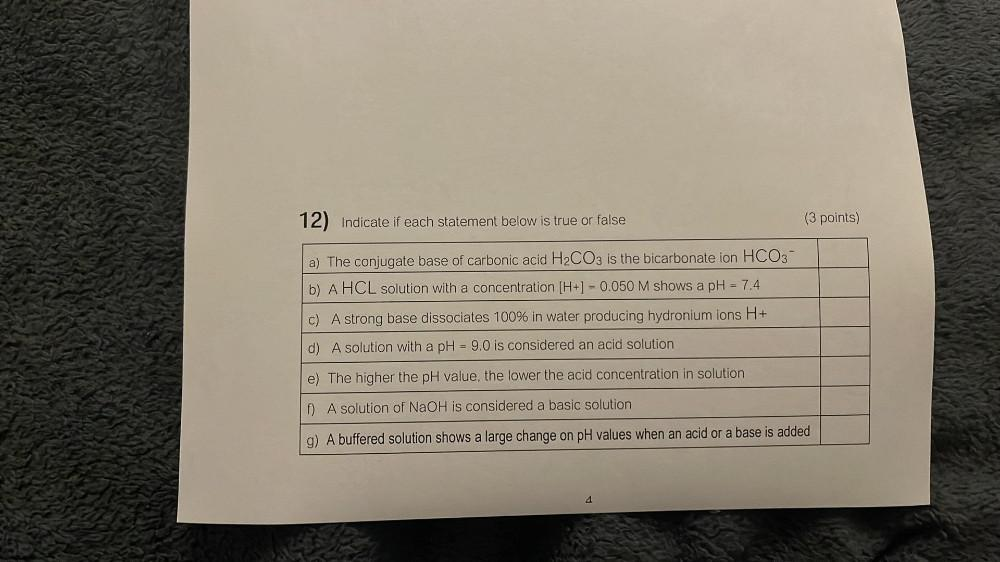Question:

# 12) Indicate if each statement below is true or false (3 points) a) The conjugate base of carbonic acid H2CO3 is the bicarbonate12) Indicate if each statement below is true or false (3 points) a) The conjugate base of carbonic acid H2CO3 is the bicarbonate ion HCO3- b) A HCL solution with a concentration [H+] = 0.050 M shows a pH = 7.4 c) A strong base dissociates 100% in water producing hydronium ions H+ d) A solution with a pH = 9.0 is considered an acid solution e) The higher the pH value, the lower the acid concentration in solution f) A solution of NaOH is considered a basic solution g) A buffered solution shows a large change on pH values when an acid or a base is added 4